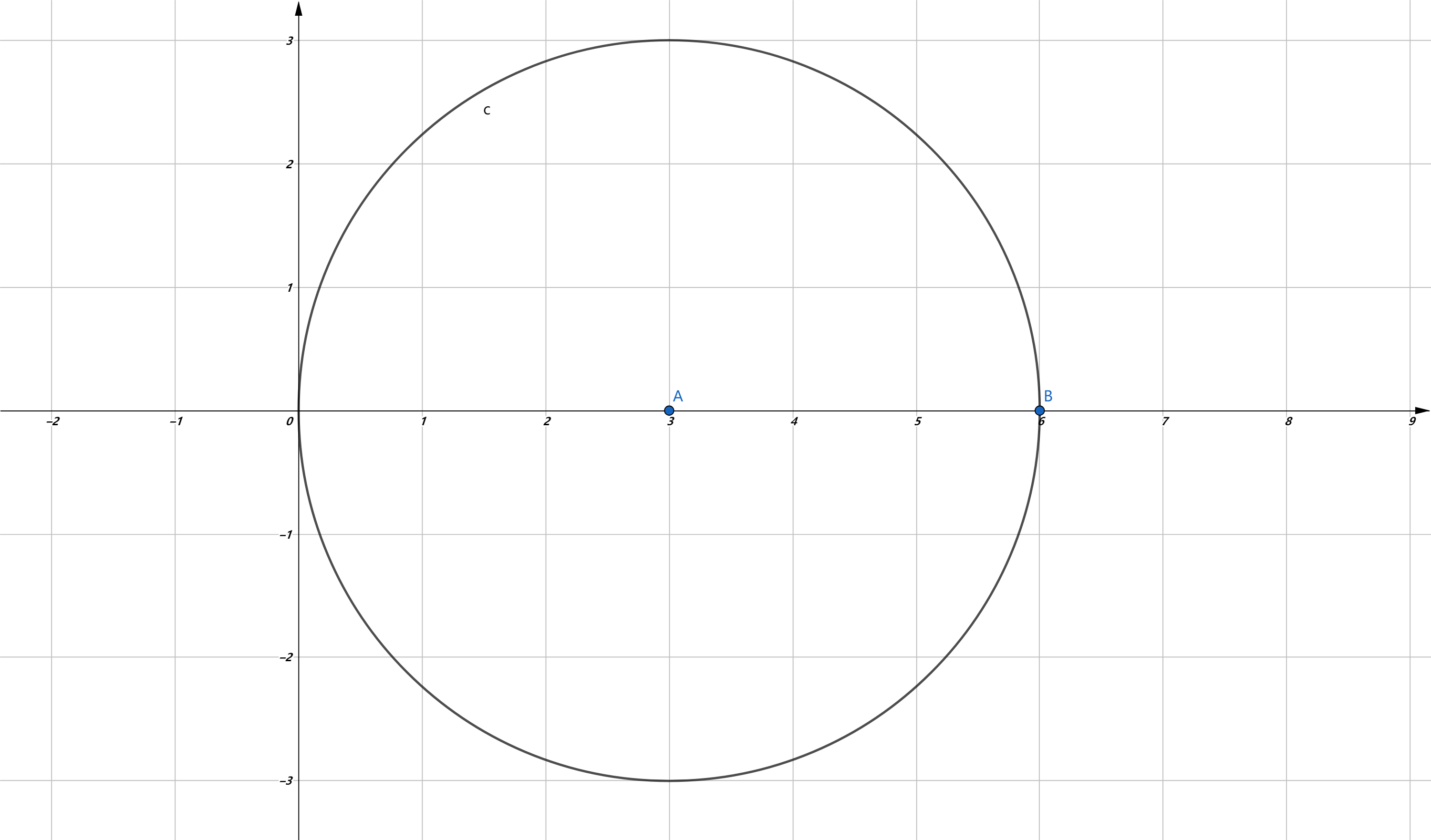安卓手机扫描二维码安装App﻿$\pi$﻿ 是数学中的明星，虽然定义为圆的周长与直径之比，但它不仅仅只出现在圆中。

﻿$\dfrac{1}{1}-\dfrac{1}{3}+\dfrac{1}{5}-\dfrac{1}{7}+\dfrac{1}{9}-\dfrac{1}{11}+\cdots=\dfrac{\pi}{4}$﻿

﻿$\dfrac{1}{1^2}+\dfrac{1}{2^2}+\dfrac{1}{3^2}+\dfrac{1}{4^2}+\dfrac{1}{5^2}+\dfrac{1}{6^2}+\cdots=\dfrac{{\pi}^2}{6}$﻿ ﻿苹果手机扫描二维码安装App